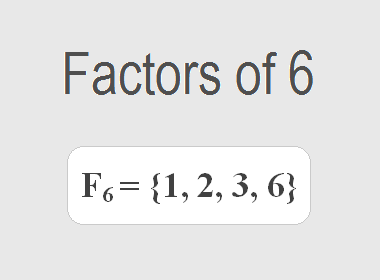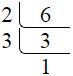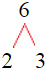# Factors of 6The factors of 6 are 1, 2, 3, and 6 i.e. F6 = {1, 2, 3, 6}. The factors of 6 are those numbers that can divide 6 without leaving a remainder.

We can check if these numbers are factors of 6 by dividing 6 by each of them. If the result is a whole number, then the number is a factor of 6. Let's do this for each of the numbers listed above:

·        1 is a factor of 6 because 6 divided by 1 is 6.

·        2 is a factor of 6 because 6 divided by 2 is 3.

·        3 is a factor of 6 because 6 divided by 3 is 2.

·        6 is a factor of 6 because 6 divided by 6 is 1.

## How to Find Factors of 6?

1 and the number itself are the factors of every number. So, 1 and 6 are two factors of 6. To find the other factors of 6, we can start by dividing 6 by the numbers between 1 and 6. If we divide 6 by 2, we get a remainder of 0. Therefore, 2 is a factor of 6. If we divide 6 by 3, we get a remainder of 0. Therefore, 3 is a factor of 6.

Next, we can check if 4 is a factor of 6. If we divide 6 by 4, we get a remainder of 2. Therefore, 4 is not a factor of 6. We can continue this process for all the possible factors of 6.

Through this process, we can find that the factors of 6 are 1, 2, 3, and 6. These are the only numbers that can divide 6 without leaving a remainder.********************

********************

## Properties of the Factors of 6

The factors of 6 have some interesting properties. One of the properties is that the sum of the factors of 6 is equal to 12. We can see this by adding all the factors of 6 together:

1 + 2 + 3 + 6 = 12

Another property of the factors of 6 is that they are all prime numbers except 1 and 6.

## Applications of the Factors of 6

The factors of 6 have several applications in mathematics. One of the applications is in finding the highest common factor (HCF) of two or more numbers. The HCF is the largest factor that two or more numbers have in common. For example, to find the HCF of 6 and 8, we need to find the factors of both numbers and identify the largest factor they have in common. The factors of 6 are 1, 2, 3, and 6. The factors of 8 are 1, 2, 4, and 8. The largest factor that they have in common is 2. Therefore, the HCF of 6 and 8 is 2.

Another application of the factors of 6 is in prime factorization. Prime factorization is the process of expressing a number as the product of its prime factors. The prime factors of 6 are 2 and 3, since these are the only prime numbers that can divide 6 without leaving a remainder. Therefore, we can express 6 as:

6 = 2 × 3

We can do prime factorization by division and factor tree method also. Here is the prime factorization of 6 by division method,6 = 2 × 3

Here is the prime factorization of 6 by the factor tree method,6 = 2 × 3

## Conclusion

The factors of 6 are the numbers that can divide 6 without leaving a remainder. The factors of 6 are 1, 2, 3, and 6. The factors of 6 have some interesting properties, such as having a sum of 12. The factors of 6 have several applications in mathematics, such as finding the highest common factor and prime factorization.